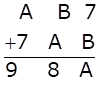Solve Cryptarithms:Asked by Sakshi | 1 year ago |  80

##### Solution :-

Firstly let us solve for unit’s place,

We have two conditions here, 7 + B ≤ 9 and 7 + B > 9

For 7 + B ≤ 9

7 + B = A

A – B = 7 (1)

Now let us solve for ten’s place,

B + A = 8  (2)

Solving 1 and 2 simultaneously,

2A = 15 which means A = 7.5 which is not possible

So, our condition 7 + B ≤ 9 is wrong.

7 + B > 9 is correct condition

Hence, there should be one carry in ten’s place and subtracting 10 from unit’s place,

7 + B – 10 = A

B – A = 3 —- (3)

For ten’s place,

B + A + 1 = 8

B + A = 8-1

B + A = 7 —- (4)

Solving (3) and (4) simultaneously,

2B = 10

B =$$\dfrac{10}{2}$$ = 5

Substituting the value of B in equation 4

B + A = 7

5 + A = 7

A = 7-5 = 2

B = 5 and A = 2

Answered by Aaryan | 1 year ago

### Related Questions

#### Which of the following numbers are divisible by 11

Which of the following numbers are divisible by 11:

(i) 10835

(ii) 380237

(iii) 504670

(iv) 28248

#### In each of the following replace * by a digit so that the number formed is divisible by 11:

In each of the following replace * by a digit so that the number formed is divisible by 11:

(i) 64 × 2456

(ii) 86 × 6194

#### In each of the following replace * by a digit so that the number formed is divisible by 6:

In each of the following replace * by a digit so that the number formed is divisible by 6:

(i) 97 × 542

(ii) 709 × 94

#### In each of the following replace × by a digit so that the number formed is divisible by 9:

In each of the following replace × by a digit so that the number formed is divisible by 9

(i) 49 × 2207

(ii) 5938 × 623